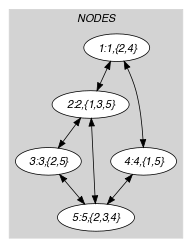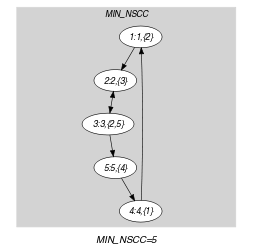## 5.381. strongly_connected

Origin
Constraint

$\mathrm{𝚜𝚝𝚛𝚘𝚗𝚐𝚕𝚢}_\mathrm{𝚌𝚘𝚗𝚗𝚎𝚌𝚝𝚎𝚍}\left(\mathrm{𝙽𝙾𝙳𝙴𝚂}\right)$

Argument
 $\mathrm{𝙽𝙾𝙳𝙴𝚂}$ $\mathrm{𝚌𝚘𝚕𝚕𝚎𝚌𝚝𝚒𝚘𝚗}\left(\mathrm{𝚒𝚗𝚍𝚎𝚡}-\mathrm{𝚒𝚗𝚝},\mathrm{𝚜𝚞𝚌𝚌}-\mathrm{𝚜𝚟𝚊𝚛}\right)$
Restrictions
 $\mathrm{𝚛𝚎𝚚𝚞𝚒𝚛𝚎𝚍}$$\left(\mathrm{𝙽𝙾𝙳𝙴𝚂},\left[\mathrm{𝚒𝚗𝚍𝚎𝚡},\mathrm{𝚜𝚞𝚌𝚌}\right]\right)$ $\mathrm{𝙽𝙾𝙳𝙴𝚂}.\mathrm{𝚒𝚗𝚍𝚎𝚡}\ge 1$ $\mathrm{𝙽𝙾𝙳𝙴𝚂}.\mathrm{𝚒𝚗𝚍𝚎𝚡}\le |\mathrm{𝙽𝙾𝙳𝙴𝚂}|$ $\mathrm{𝚍𝚒𝚜𝚝𝚒𝚗𝚌𝚝}$$\left(\mathrm{𝙽𝙾𝙳𝙴𝚂},\mathrm{𝚒𝚗𝚍𝚎𝚡}\right)$
Purpose

Consider a digraph $G$ described by the $\mathrm{𝙽𝙾𝙳𝙴𝚂}$ collection. Select a subset of arcs of $G$ so that we have a single strongly connected component involving all vertices of $G$.

Example
$\left(\begin{array}{c}〈\begin{array}{cc}\mathrm{𝚒𝚗𝚍𝚎𝚡}-1\hfill & \mathrm{𝚜𝚞𝚌𝚌}-\left\{2\right\},\hfill \\ \mathrm{𝚒𝚗𝚍𝚎𝚡}-2\hfill & \mathrm{𝚜𝚞𝚌𝚌}-\left\{3\right\},\hfill \\ \mathrm{𝚒𝚗𝚍𝚎𝚡}-3\hfill & \mathrm{𝚜𝚞𝚌𝚌}-\left\{2,5\right\},\hfill \\ \mathrm{𝚒𝚗𝚍𝚎𝚡}-4\hfill & \mathrm{𝚜𝚞𝚌𝚌}-\left\{1\right\},\hfill \\ \mathrm{𝚒𝚗𝚍𝚎𝚡}-5\hfill & \mathrm{𝚜𝚞𝚌𝚌}-\left\{4\right\}\hfill \end{array}〉\hfill \end{array}\right)$

The $\mathrm{𝚜𝚝𝚛𝚘𝚗𝚐𝚕𝚢}_\mathrm{𝚌𝚘𝚗𝚗𝚎𝚌𝚝𝚎𝚍}$ constraint holds since the $\mathrm{𝙽𝙾𝙳𝙴𝚂}$ collection depicts a graph involving a single strongly connected component (i.e., since we have a circuit visiting successively the vertices 1, 2, 3, 5, and 4).

Typical
$|\mathrm{𝙽𝙾𝙳𝙴𝚂}|>2$
Symmetry

Items of $\mathrm{𝙽𝙾𝙳𝙴𝚂}$ are permutable.

Algorithm

The sketch of a filtering algorithm for the $\mathrm{𝚜𝚝𝚛𝚘𝚗𝚐𝚕𝚢}_\mathrm{𝚌𝚘𝚗𝚗𝚎𝚌𝚝𝚎𝚍}$ constraint is given in [Dooms06].

related: $\mathrm{𝚌𝚒𝚛𝚌𝚞𝚒𝚝}$ (one single strongly connected component in the final solution).

Keywords
Arc input(s)

$\mathrm{𝙽𝙾𝙳𝙴𝚂}$

Arc generator
$\mathrm{𝐶𝐿𝐼𝑄𝑈𝐸}$$↦\mathrm{𝚌𝚘𝚕𝚕𝚎𝚌𝚝𝚒𝚘𝚗}\left(\mathrm{𝚗𝚘𝚍𝚎𝚜}\mathtt{1},\mathrm{𝚗𝚘𝚍𝚎𝚜}\mathtt{2}\right)$

Arc arity
Arc constraint(s)
$\mathrm{𝚒𝚗}_\mathrm{𝚜𝚎𝚝}$$\left(\mathrm{𝚗𝚘𝚍𝚎𝚜}\mathtt{2}.\mathrm{𝚒𝚗𝚍𝚎𝚡},\mathrm{𝚗𝚘𝚍𝚎𝚜}\mathtt{1}.\mathrm{𝚜𝚞𝚌𝚌}\right)$
Graph property(ies)
$\mathrm{𝐌𝐈𝐍}_\mathrm{𝐍𝐒𝐂𝐂}$$=|\mathrm{𝙽𝙾𝙳𝙴𝚂}|$

Graph model

Part (A) of Figure 5.381.1 shows the initial graph from which we start. It is derived from the set associated with each vertex. Each set describes the potential values of the $\mathrm{𝚜𝚞𝚌𝚌}$ attribute of a given vertex. Part (B) of Figure 5.381.1 gives the final graph associated with the Example slot. The $\mathrm{𝚜𝚝𝚛𝚘𝚗𝚐𝚕𝚢}_\mathrm{𝚌𝚘𝚗𝚗𝚎𝚌𝚝𝚎𝚍}$ constraint holds since the final graph contains a single strongly connected component mentioning every vertex of the initial graph.

##### Figure 5.381.1. Initial and final graph of the $\mathrm{𝚜𝚝𝚛𝚘𝚗𝚐𝚕𝚢}_\mathrm{𝚌𝚘𝚗𝚗𝚎𝚌𝚝𝚎𝚍}$ set constraint(a) (b)
Signature

Since the maximum number of vertices of the final graph is equal to $|\mathrm{𝙽𝙾𝙳𝙴𝚂}|$ we can rewrite the graph property $\mathrm{𝐌𝐈𝐍}_\mathrm{𝐍𝐒𝐂𝐂}=|\mathrm{𝙽𝙾𝙳𝙴𝚂}|$ to $\mathrm{𝐌𝐈𝐍}_\mathrm{𝐍𝐒𝐂𝐂}\ge |\mathrm{𝙽𝙾𝙳𝙴𝚂}|$ and simplify $\underline{\overline{\mathrm{𝐌𝐈𝐍}_\mathrm{𝐍𝐒𝐂𝐂}}}$ to $\overline{\mathrm{𝐌𝐈𝐍}_\mathrm{𝐍𝐒𝐂𝐂}}$.